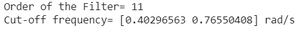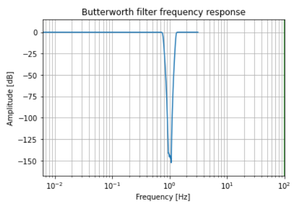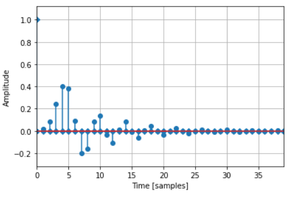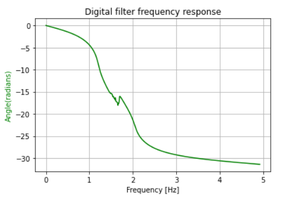# Digital Band Reject Butterworth Filter in Python

• Last Updated : 03 Jan, 2021

In this article, we are going to discuss how to design a Digital Band Reject Butterworth Filter using Python. The Butterworth filter is a type of signal processing filter designed to have a frequency response as flat as possible in the pass band. Let us take the below specifications to design the filter and observe the Magnitude, Phase & Impulse Response of the Digital Butterworth Filter.

## What is Digital Bandreject Filter?

A band-pass filter is a filter that passes frequencies within a range and rejects frequencies outside that range.

## How it’s different from Highpass, Lowpass & Bandpass:

The main difference can be spotted by observing the magnitude response of the Band Pass Filter. In the Band-Reject filter, all the signals between the specified frequency range get rejected by the filter.

The specifications are as follows:

• The sampling rate of 12 kHz.
• Pass band edge frequencies are 2100 Hz & 4500 Hz.
• Stop band edge frequencies are 2700 Hz & 3900 Hz.
• Pass band ripple of 0.6 dB.
• Minimum stop band attenuation of 45 dB.

We will plot the magnitude, phase, and impulse response of the filter.

### Step-by-step Approach:

Before starting, first, we will create a user-defined function to convert the edge frequencies, we are defining it as the convert() method.

## Python3

 `# explicit function to convert ` `# edge frequencies ` ` `  ` `  `def` `convertX(f_sample, f): ` `    ``w ``=` `[] ` ` `  `    ``for` `i ``in` `range``(``len``(f)): ` `        ``b ``=` `2``*``((f[i]``/``2``) ``/` `(f_sample``/``2``)) ` `        ``w.append(b) ` ` `  `    ``omega_mine ``=` `[] ` ` `  `    ``for` `i ``in` `range``(``len``(w)): ` `        ``c ``=` `(``2``/``Td)``*``np.tan(w[i]``/``2``) ` `        ``omega_mine.append(c) ` ` `  `    ``return` `omega_mine `

Step 1: Importing all the necessary libraries.

## Python3

 `# import required modules ` `import` `numpy as np ` `import` `matplotlib.pyplot as plt ` `from` `scipy ``import` `signal ` `import` `math `

Step 2: Define variables with the given specifications of the filter.

## Python3

 `# Specifications of Filter ` ` `  `# sampling frequency ` `f_sample ``=` `12000` ` `  `# pass band frequency ` `f_pass ``=` `[``2100``, ``4500``] ` ` `  `# stop band frequency ` `f_stop ``=` `[``2700``, ``3900``] ` ` `  `# pass band ripple ` `fs ``=` `0.5` ` `  `# Sampling Time ` `Td ``=` `1` ` `  `# pass band ripple ` `g_pass ``=` `0.6` ` `  `# stop band attenuation ` `g_stop ``=` `45`

Step 3: Building the filter using signal.buttord() function.

## Python3

 `# Conversion to prewrapped analog ` `# frequency ` `omega_p ``=` `convertX(f_sample, f_pass) ` `omega_s ``=` `convertX(f_sample, f_stop) ` ` `  `# Design of Filter using signal.buttord ` `# function ` `N, Wn ``=` `signal.buttord(omega_p, omega_s, ` `                       ``g_pass, g_stop, ` `                       ``analog``=``True``) ` ` `  ` `  `# Printing the values of order & cut-off frequency ` `# N is the order ` `print``(``"Order of the Filter="``, N) ` ` `  `# Wn is the cut-off freq of the filter ` `print``(``"Cut-off frequency= {:} rad/s "``.``format``(Wn)) ` ` `  ` `  `# Conversion in Z-domain ` ` `  `# b is the numerator of the filter & a is ` `# the denominator ` `b, a ``=` `signal.butter(N, Wn, ``'bandpass'``, ``True``) ` `z, p ``=` `signal.bilinear(b, a, fs) ` ` `  `# w is the freq in z-domain & h is the ` `# magnitude in z-domain ` `w, h ``=` `signal.freqz(z, p, ``512``) `

Output:Step 4: Plotting the Magnitude Response.

## Python3

 `# Magnitude Response ` `plt.semilogx(w, ``20``*``np.log10(``abs``(h))) ` `plt.xscale(``'log'``) ` `plt.title(``'Butterworth filter frequency response'``) ` `plt.xlabel(``'Frequency [Hz]'``) ` `plt.ylabel(``'Amplitude [dB]'``) ` `plt.margins(``0``, ``0.1``) ` `plt.grid(which``=``'both'``, axis``=``'both'``) ` `plt.axvline(``100``, color``=``'green'``) ` `plt.show() `

Output:Step 5: Plotting the Impulse Response.

## Python3

 `# Impulse Response ` `imp ``=` `signal.unit_impulse(``40``) ` `c, d ``=` `signal.butter(N, ``0.5``) ` `response ``=` `signal.lfilter(c, d, imp) ` ` `  `plt.stem(np.arange(``0``, ``40``), imp, markerfmt``=``'D'``, use_line_collection``=``True``) ` `plt.stem(np.arange(``0``, ``40``), response, use_line_collection``=``True``) ` `plt.margins(``0``, ``0.1``) ` ` `  `plt.xlabel(``'Time [samples]'``) ` `plt.ylabel(``'Amplitude'``) ` `plt.grid(``True``) ` `plt.show() `

Output:Step 6: Plotting the Phase Response.

## Python3

 `# Frequency Response ` `fig, ax1 ``=` `plt.subplots() ` `ax1.set_title(``'Digital filter frequency response'``) ` `ax1.set_ylabel(``'Angle(radians)'``, color``=``'g'``) ` `ax1.set_xlabel(``'Frequency [Hz]'``) ` ` `  `angles ``=` `np.unwrap(np.angle(h)) ` ` `  `ax1.plot(w``/``2``*``np.pi, angles, ``'g'``) ` `ax1.grid() ` `ax1.axis(``'tight'``) ` `plt.show() `

Output:Below is the complete program based on the above approach:

## Python3

 `# import required modules ` `import` `numpy as np ` `import` `matplotlib.pyplot as plt ` `from` `scipy ``import` `signal ` `import` `math ` ` `  `# explicit function to convert ` `# edge frequencies ` `def` `convertX(f_sample, f): ` `    ``w ``=` `[] ` ` `  `    ``for` `i ``in` `range``(``len``(f)): ` `        ``b ``=` `2``*``((f[i]``/``2``)``/``(f_sample``/``2``)) ` `        ``w.append(b) ` ` `  `    ``omega_mine ``=` `[] ` ` `  `    ``for` `i ``in` `range``(``len``(w)): ` `        ``c ``=` `(``2``/``Td)``*``np.tan(w[i]``/``2``) ` `        ``omega_mine.append(c) ` ` `  `    ``return` `omega_mine ` ` `  `# Specifications of Filter ` `# sampling frequency ` `f_sample ``=` `12000` ` `  `# pass band frequency ` `f_pass ``=` `[``2100``, ``4500``] ` ` `  `# stop band frequency ` `f_stop ``=` `[``2700``, ``3900``] ` ` `  `# pass band ripple ` `fs ``=` `0.5` ` `  `# Sampling Time ` `Td ``=` `1` ` `  `# pass band ripple ` `g_pass ``=` `0.6` ` `  `# stop band attenuation ` `g_stop ``=` `45` ` `  `# Conversion to prewrapped analog ` `# frequency ` `omega_p ``=` `convertX(f_sample, f_pass) ` `omega_s ``=` `convertX(f_sample, f_stop) ` ` `  `# Design of Filter using signal.buttord ` `# function ` `N, Wn ``=` `signal.buttord(omega_p, omega_s, g_pass, ` `                       ``g_stop, analog``=``True``) ` ` `  `# Printing the values of order & cut-off frequency ` `# N is the order ` `print``(``"Order of the Filter="``, N) ` ` `  `# Wn is the cut-off freq of the filter ` `print``(``"Cut-off frequency= {:} rad/s "``.``format``(Wn)) ` ` `  ` `  `# Conversion in Z-domain ` ` `  `# b is the numerator of the filter & a is ` `# the denominator ` `b, a ``=` `signal.butter(N, Wn, ``'bandpass'``, ``True``) ` `z, p ``=` `signal.bilinear(b, a, fs) ` ` `  `# w is the freq in z-domain & h is the ` `# magnitude in z-domain ` `w, h ``=` `signal.freqz(z, p, ``512``) ` ` `  `# Magnitude Response ` `plt.semilogx(w, ``20``*``np.log10(``abs``(h))) ` `plt.xscale(``'log'``) ` `plt.title(``'Butterworth filter frequency response'``) ` `plt.xlabel(``'Frequency [Hz]'``) ` `plt.ylabel(``'Amplitude [dB]'``) ` `plt.margins(``0``, ``0.1``) ` `plt.grid(which``=``'both'``, axis``=``'both'``) ` `plt.axvline(``100``, color``=``'green'``) ` `plt.show() ` ` `  `# Impulse Response ` `imp ``=` `signal.unit_impulse(``40``) ` `c, d ``=` `signal.butter(N, ``0.5``) ` `response ``=` `signal.lfilter(c, d, imp) ` ` `  `plt.stem(np.arange(``0``, ``40``), imp, ` `         ``markerfmt``=``'D'``, ` `         ``use_line_collection``=``True``) ` ` `  `plt.stem(np.arange(``0``, ``40``), response, ` `         ``use_line_collection``=``True``) ` ` `  `plt.margins(``0``, ``0.1``) ` ` `  `plt.xlabel(``'Time [samples]'``) ` `plt.ylabel(``'Amplitude'``) ` `plt.grid(``True``) ` `plt.show() ` ` `  `# Frequency Response ` `fig, ax1 ``=` `plt.subplots() ` `ax1.set_title(``'Digital filter frequency response'``) ` `ax1.set_ylabel(``'Angle(radians)'``, color``=``'g'``) ` `ax1.set_xlabel(``'Frequency [Hz]'``) ` ` `  `angles ``=` `np.unwrap(np.angle(h)) ` ` `  `ax1.plot(w``/``2``*``np.pi, angles, ``'g'``) ` `ax1.grid() ` `ax1.axis(``'tight'``) ` `plt.show() `

Output:My Personal Notes arrow_drop_up
Recommended Articles
Page :